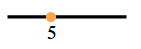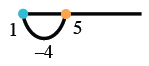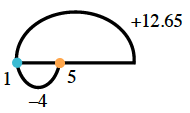### Home > CC1MN > Chapter 4 Unit 4 > Lesson cc14.1.1 > Problem4-9

4-9.

Simplify each expression below. For each expression, draw a picture or show how you know your answer makes sense.

1. $5+(−4)+12.65$

• Use a drawing such as the one to the right to help you make sense of the problem.

• Starting at $5$, add $−4$. In other words, subtract 4.

• Now add $12.65$.

• $13.65$1. $6.5+(−2)+10.5$

• See part (a).

1. $4(−5+100)$

Add what is in the parentheses first, then multiply by 4.

2. $−212+(−102)$

Use a drawing as in part (a) to help make sense of this problem.

3. $4+6(3)+2(5\frac{1}{2}−1)$

Remember to subtract what is in the parentheses first before multiplying by 2.

Make sure all multiplication is done before adding.

$31$

4. $5+3(5)+(−4)(5)$